# Introduction to Trees

Tree is a discrete structure that represents hierarchical relationships between individual elements or nodes. A tree in which a parent has no more than two children is called a binary tree.

## Tree and its Properties

Definition − A Tree is a connected acyclic undirected graph. There is a unique path between every pair of vertices in $G$. A tree with N number of vertices contains $(N-1)$ number of edges. The vertex which is of 0 degree is called root of the tree. The vertex which is of 1 degree is called leaf node of the tree and the degree of an internal node is at least 2.

Example − The following is an example of a tree −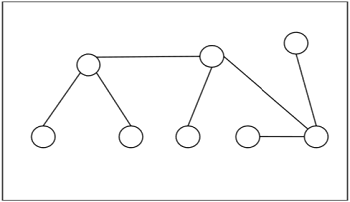## Centers and Bi-Centers of a Tree

The center of a tree is a vertex with minimal eccentricity. The eccentricity of a vertex $X$ in a tree $G$ is the maximum distance between the vertex $X$ and any other vertex of the tree. The maximum eccentricity is the tree diameter. If a tree has only one center, it is called Central Tree and if a tree has only more than one centers, it is called Bi-central Tree. Every tree is either central or bi-central.

### Algorithm to find centers and bi-centers of a tree

Step 1 − Remove all the vertices of degree 1 from the given tree and also remove their incident edges.

Step 2 − Repeat step 1 until either a single vertex or two vertices joined by an edge is left. If a single vertex is left then it is the center of the tree and if two vertices joined by an edge is left then it is the bi-center of the tree.

Problem 1

Find out the center/bi-center of the following tree −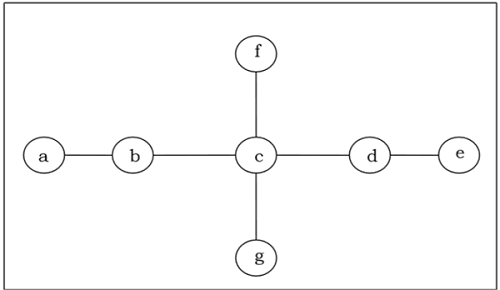Solution

At first, we will remove all vertices of degree 1 and also remove their incident edges and get the following tree −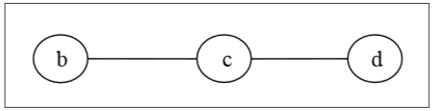Again, we will remove all vertices of degree 1 and also remove their incident edges and get the following tree −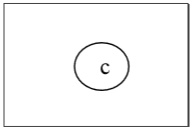Finally we got a single vertex ‘c’ and we stop the algorithm. As there is single vertex, this tree has one center ‘c’ and the tree is a central tree.

Problem 2

Find out the center/bi-center of the following tree −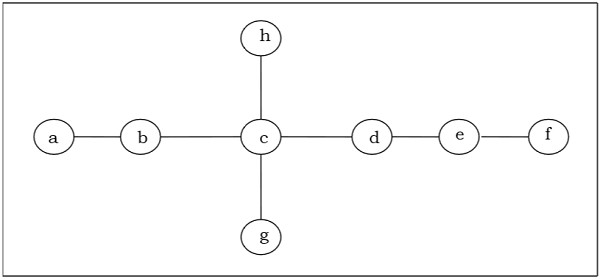Solution

At first, we will remove all vertices of degree 1 and also remove their incident edges and get the following tree −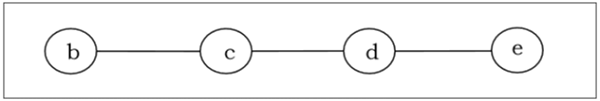Again, we will remove all vertices of degree 1 and also remove their incident edges and get the following tree −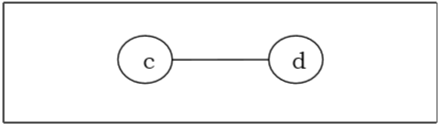Finally, we got two vertices ‘c’ and ‘d’ left, hence we stop the algorithm. As two vertices joined by an edge is left, this tree has bi-center ‘cd’ and the tree is bi-central.

## Labeled Trees

Definition − A labeled tree is a tree the vertices of which are assigned unique numbers from 1 to n. We can count such trees for small values of n by hand so as to conjecture a general formula. The number of labeled trees of n number of vertices is $n^{n-2}$. Two labeled trees are isomorphic if their graphs are isomorphic and the corresponding points of the two trees have the same labels.

### Example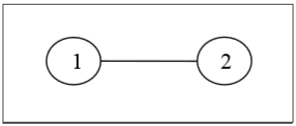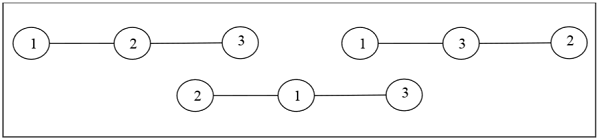## Unlabeled Trees

Definition − An unlabeled tree is a tree the vertices of which are not assigned any numbers. The number of labeled trees of n number of vertices is $\frac {(2n)!}{ (n+1)!n! }$ (nth Catalan number)

### Example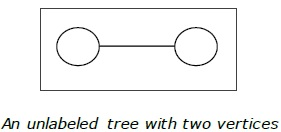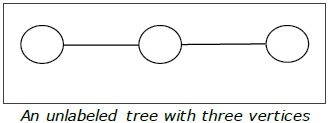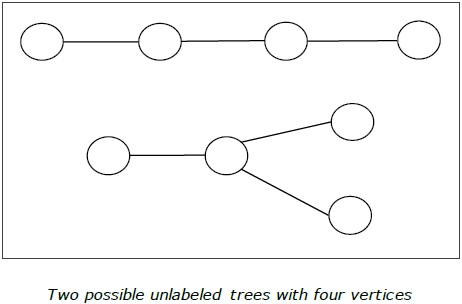## Rooted Tree

A rooted tree $G$ is a connected acyclic graph with a special node that is called the root of the tree and every edge directly or indirectly originates from the root. An ordered rooted tree is a rooted tree where the children of each internal vertex are ordered. If every internal vertex of a rooted tree has not more than m children, it is called an m-ary tree. If every internal vertex of a rooted tree has exactly m children, it is called a full m-ary tree. If $m = 2$, the rooted tree is called a binary tree.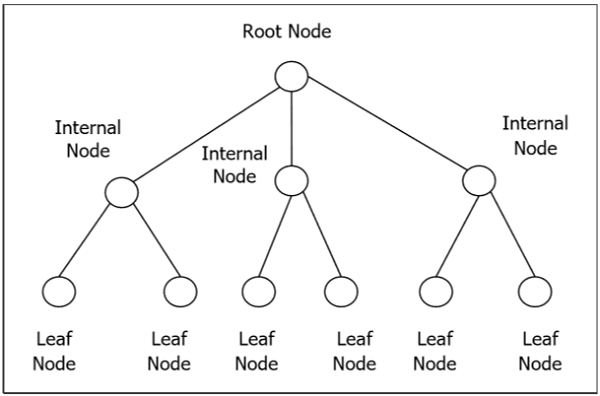## Binary Search Tree

Binary Search tree is a binary tree which satisfies the following property −

• $X$ in left sub-tree of vertex $V, Value(X) \le Value (V)$
• $Y$ in right sub-tree of vertex $V, Value(Y) \ge Value (V)$

So, the value of all the vertices of the left sub-tree of an internal node $V$ are less than or equal to $V$ and the value of all the vertices of the right sub-tree of the internal node $V$ are greater than or equal to $V$. The number of links from the root node to the deepest node is the height of the Binary Search Tree.

### Example### Algorithm to search for a key in BST

BST_Search(x, k)
if ( x = NIL or k = Value[x] )
return x;
if ( k < Value[x])
return BST_Search (left[x], k);
else
return BST_Search (right[x], k)


### Complexity of Binary search tree

Average Case Worst case
Space Complexity O(n) O(n)
Search Complexity O(log n) O(n)
Insertion Complexity O(log n) O(n)
Deletion Complexity O(log n) O(n)